## BREAK NEWS# Pressure in liquids, density, viscosity, Bernoulli’s principle## Pressure in liquids

This is the force exerted normally per unit area. The SI units of pressure of liquids are Nm-2 or Pascals (pa)The pressure in liquids is independent of the shape and cross sectional are as shown below

Volume  of a liquid displaced = Ah (A = cross section area)

Mass of the liquid displaced  = Ahρ (ρ = density of a liquid)

Weight of the liquid displaced = Ahρg (g = acceleration due to gravity)Floating objects

Archimedes principle

When a body is partially or fully immersed in a fluid, it experiences an upthrust which is equal to the weight of a fluid displaced.

Consider  a solid of cross section area A immersed in a liquid of density,Total pressure at the top = H + h1ρg

Force on top surface = (H + h1ρg)A

Total pressure at the bottom = H + h2ρg

Force on bottom surface = (H + h2ρg)A

Resultant upward force = upthrust

= (H + h2ρg)A – (H + h1ρg)A

= (h2 – h1) ρgA

But (h2 – h1) A   = volume of the solid

= volume of the liquid displaced

(h2 – h1) Aρg  = weight of liquid displaced

Upthrust  = weight of the liquid displaced

Conclusion

Since there is no side way movement, the resultant horizontal force is zero. Therefore, up thrust is equal to the volume of fluid displaced.

Law of floatation

States that a floating object displaces a liquid equal to its own weight of the liquid in which it floats

Example 1

A string supports a solid of mass 5kg totally immersed in a liquid of density 800kgm-3. Find the tension in the string if the object has a density of 2575kgm-3.

SolutionExample 2

A piece of metal of mass 2.60 x 10-3 kg and density 8.4 x 103kgm-3 is attached to the block of mass of 1.0x 10-2kg and density 9.2 x 102kgm-3. When the system is placed in a fluid, it floats with wax just submerged. Find the density of the fluid.Example 3

A solid of density, ρ, floats at the interface of two liquids of densities ρ1 and ρ2 with 80% of its volume in liquid of density ρ1. Show that )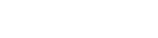Relative density (R.D)

This is the ratio of mass of a substance to the mass of equal volume of water.Example 4

A solid of mass 0.2kg is suspended from a spring balance when the block is immersed in water the spring reads 0.84N. When the block is immersed in a liquid of unknown density, the spring balances reads 0.95N. Find

• Density of the block
• Density of the liquidExperiment to determine the relative density of a substance that floats in water

Apparatus: thread, spring balance, object, sinker and water

By means of a thread tied to the object, determine the weight, W1, of an object in air using a spring balance.

Attach a sinker to the object and immerse the two in water and determine the weight, W2, of the body and the sinker.

Determine the weight of the sinker, W3.Density of the substance,  = R.D x 1000kgm-3

Experiment to determine the relative density of a liquid

By means of a thread, determine the weight of solid in air, liquid, and water = W1, W2, and W3 respectively.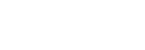## Density rod

This is a rod used to compare densities of two liquids. The higher the rod floats, the higher the density of the liquid. A density rod floats with height h1, submerged in water. In oil, it floats with height, h2, submerged. Show that the relative density of oil is .

SolutionMass of the rod = mass of water displaced = mass of oil displaced

Let the rod have a cross sectional area = A

Ah1ρw = Ah2ρo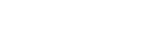Example 5

An object of mass 30g and density 2g/cm3 has a uniform cross section area of 3cm2 floats in water and oil leaving a height of 1.5 and 0.5cm respectively above the surfaces. Calculate the relative density of oil.SolutionHygrometer

It consists of a bulb that floats upright above a liquid surface. Lead shots are placed in the bulb to ensure that the system floats upright.

Example 6

A hygrometer floats on water with 72% of its volume submerged in a liquid. It floats with 68% of its volume submerged. Find the relative density of the liquid

Mass of hygrometer = mass of water displaced = mass of liquid displaced

Let the volume of hygrometer = V## Types of flow

1. Laminar flow

Laminar flow occurs when the fluid flows in infinitesimal parallel layers with no disruption between them. The successive particles passing a given point have the same velocity.

The velocity of particles may change from one streamline to another

1. Turbulence/turbulent flow/non uniform flow

In turbulent flow the speed of the fluid at a point is continuously undergoing changes in both magnitude and direction.

Common examples of turbulent flow are blood flow in arteries, oil transport in pipelines, lava flow, atmosphere and ocean currents, the flow through pumps and turbines, and the flow in boat wakes and around aircraft-wing tips.

Experiment to demonstrate laminar and turbulent flowWater is kept flowing at a constant velocity from a constant water tank.

The rate of flow of a dye is controlled by a tap A.

At low water velocity a streamline of a dye is observed flowing through water. This is laminar flow

A turbulent flow is observed when the velocity of water is increased here the dye mixes with water.

Viscosity

This is the frictional force that opposes the relative motion between different fluid layers.  It is the result of inter molecular forces between particles within a fluid which necessitates work to be done when layer move over one another.

Factors affecting the magnitude of viscosity

1. Temperature: increase in temperature reduces inter-molecular forces due to increased kinetic energy. This reduces viscosity.

In gases viscosity increases as temperature increases due to molecular diffusion from one layer to another of different velocities. As the temperature increases, the rate of diffusion also increases and the drag exerted on each layer by the other increases.

1. Chemical composition

The viscosity of liquids generally depends upon the size, shape and chemical nature of their mol­ecules.

It is greater with larger than with smaller molecules; with elongated than with spherical molecules.

Large amounts of dissolved solids generally increase vis­cosity. Small amounts of electrolytes lower the viscosity of water slightly.

1. Colloid Systems:

The viscosity of lyophilic colloid solution is generally relatively high.

1. Suspended Material:

Suspended particles cause an increase in the viscosity. The viscosity of blood is important in relation to the resistance offered to the heart in circulating the blood. The heart muscle functions best while working against a certain resistance. The viscosity of blood is due largely to the emulsoid colloid system present in plasma and to the great proportion of suspended corpuscles.

This is the change in velocity per unit length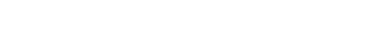Newton’s law of viscosity

The frictional force between different fluid layers is directly proportional to the area of molecular layer.If A = 1m2,  = 1s-1, F = 1N

ղ = 1Nsm-2

Coefficient of viscosity of a liquid

This is the frictional force per unit area exerted on the fluid in the region of a unit velocity gradient

Or

It is the ratio of tangential stress exerted on layers of fluid to velocity gradient. Units are NM-2s or Ns/m2. Other units are kgm-1s-1.

Example 7

A metal plate of area 0.25m2 is connected to 8g mass via a light string that passes over a frictionless pulley. A lubricant with a film of thickness 0.6mm is placed between the plate and the horizontal surface. When released, the plate moves with a speed of 87ms-1

(i) Find the coefficient of viscosity of lubricant

SolutionF = mg= 2.16 x 10-3Nsm-2

(ii) the top layer of the film is assumed to move with the same velocity as the metal plate while the bottom layer is stationary.

Viscous drag

This is the frictional force that oppose relative motion between a solid and a viscous fluid

Stokes’ law

The viscous drag experienced by an object depends on the velocity, viscosity constant and the radius of an object.For M: y = 1

For T: -2 = -x – y

x = 1

For L: 1 = x – y +z

Z = 1

From repeated experiments, k = 6π

F = 6πղvr

Example 8

The water drop of mass 10g falls through air of viscosity constant 1.0x 10-5Pa. Calculate the viscous drag, experienced by the droplet when it attains a terminal velocity of 2mms-1

Solution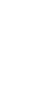F = 6πղvr

m = volume x densityr = 0.0134m

F = 6πղvr = 6π x (1.0 x10-5) x (2 x 10-3) x 0.0134

= 5.04 x10-4N

### Terminal velocity

Consider a spherical object dropped in a viscous fluid

As the object drops, it is acted on by three forces, U= up thrust up, viscous drag up and weight, (mg) down.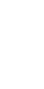F = mg – (U+v)

But U = 6πղv0r

As the velocity increases, the viscous drag force increases. At a certain velocity v0, known as terminal velocity, the resultant force acting at the body is zero.

mg = U + v

### Definition

Terminal velocity is the maximum constant velocity attained by an object falling through a viscous fluid.

A graph of the velocity of an object falling through a viscous fluid against timeExample 9

Explain why raindrops hit the ground with less force than they should.

Solution

The drag force and up thrust reduce the force by which raindrops would hit the grounds

Derivation of terminal velocity

Consider a spherical object of radius r and density, σ, falling through a viscous fluid of density, ρ, and viscous constant, ղ.mg  = U + vExample 10

A spherical ball of radius 2.5mm and density 900kgm-3 fall through air of viscosity constant 1.88 x 10-3.calculate the terminal velocity if

• Density of air is 1.29kgm-3.
• Density of air is negligible.Example 11

Eight similar water drops fall with a terminal velocity of 5mms-1. And when mid-way, they coalesce forming a big droplet. Calculate the terminal velocity of a big droplet if the density of air is negligible.

Solution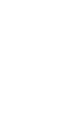Example 12

A metallic ball of mass 0.9g and diameter 8mm is dropped in oil of density 780kgm-3 attaining a terminal velocity of 0.07ms-1. A ball falls with terminal velocity of 0.03ms-1 when oil is replaced with water of density 1000kgm-3. Find the ratio of the coefficient of viscosity of oil to that of water at the same temperature.

SolutionExperiment to determine the coefficient of viscosity of a viscous fluid such as heavy oil1. A viscous fluid of density, ρ, at constant temperature is put in a tall glass jar with reference marks P and Q a distance, S, apart.
2. A ball bearing of density, σ, and radius, r, is dropped into the fluid.
3. Time taken, t, taken for the ball bearing to drop from P to Q is noted
4. Assuming the ball bearing travels with a terminal velocity, v, between P and Q, thenExperiment to determine the coefficient of viscosity of a viscous liquid using the graphical method

1. A viscous fluid of density, ρ, at constant temperature is put in a tall glass jar with reference marks P and Q a distance, S, apart.
2. A ball bearing of density, σ, and radius, r, is dropped into the fluid.
3. Time taken, t, taken for the ball bearing to drop from P to Q is noted
4. Assuming the ball bearing travels with a terminal velocity, v, between P and Q, then, v0 =
5. The procedure is repeated for different ball bearing having various radii.
6. The results of t, r, vo, r2 are tabulated.
7. A graph of v0 against r2 is plotted.Experiment to compare the coefficient of viscosity of two viscous fluids

1. A viscous fluid 1 of density, ρ1, at constant temperature is put in a tall glass jar with reference marks P and Q a distance, S, apart.
2. A ball bearing of density, σ, and radius, r, is dropped into the fluid.
3. Time taken, t1, taken for the ball bearing to drop from P to Q is noted
4. Assuming the ball bearing travels with a terminal velocity, v0, between P and Q, then,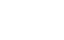5. Procedure 1, 2, 3, 4 are repeated for viscous fluid 2 of density ρ2 and time t2 to fall from P to Q is determined## Poissulle’s law

During steady flow, the rate of flow of a liquid through a pipe depends on;

(i) Coefficient of viscosity of the fluid

(iii) The pressure gradient across the pipeThree pipes are arranged in some area as shown above. If the pressure in the first pipe is P1, deduce the pressure in the second and third  pipe assuming there is a steady flow and 2l1 = 3l2 = ½ l3

Solution### Experiment to determine coefficient of viscosity of non-viscous liquid

1. One end of capillary tube whose diameter, r, is known is connected to constant pressure apparatus
2. The liquid is allowed to flow in a capillary tube until a steady state is reached when height, H, is stable
3. Volume of liquid V flowing out in time t is measured.
4. The pressure height, h, in the capillary tube is measured.Incompressible liquid

This is the liquid whose density does not change with change in pressure.

Continuity equation

Consider a non-viscous incompressible liquid flowing through a tube of non-uniform cross sectional area.Volume of a liquid between P and Q = volume of a liquid between R and SLiquids travel longer distances with high velocity in pipes of small diameter compared to those of large diameters.For a streamline, the velocity of a liquid at any section of the pipe is inversely proportional to the area of cross section at that point

## Bernoulli’s principle

For a streamline flow, the sum of pressure, kinetic energy per unit volume and potential energy per unit volume is constant at all points for a non-viscous incompressible fluid.

Bernoulli’s equation

A moving liquid has 3 types of energies

1. Kinetic energy: energy possessed by a liquid due to motion
2. Potential energy: energy possessed by a liquid due to its position in the field of force
3. Pressure energy: energy posed by a liquid due to its pressure at particular point.Example 14

Explain why Bernoulli’s equation only applies for a non-viscous incompressible fluid.

Solution

For the viscous fluid, energy is not constant while for compressible liquids, the density keeps on changing.

Example 15

Water enters a house through a supply pipe of diameter 2cm at a velocity of 0.1ms-1. The internal house connection pipe has the diameter of 1.0cm.

Calculate

(i) Speed of water as it enters the house

(ii)The rate of mass flow of water it it has a density of 1000kgm-3.

Solution

(i) A1V1 = A2V2

π(0.01)2 x 0.1 = π(0.00)2V2

V2 = 0.4ms-1

(ii) Volume per second = AV

Mass per second     = AVρ

= π(0.01)2 x 0.1 x 1000

= 0.0314kgs-1

Example 16

A compound sprinkler has 8 holes each of cross sectional area of 0.05cm2 is connected to a supply pipe of area 2.5cm2. If the speed of water in the pipe is 4ms-1, calculate the speed with which water jets out of the sprinkler into the grass.

Solution

A1V1 = A2V2

(2.5 x 10-4) x 4 = 8 (0.05 x 10-4)V2

V2 = 25ms-1

Example 17

Water flows along a horizontal pipe of cross sectional area 48cm2 with a pressure of 105Pa. The pipe has a constriction of area 12cm2 at one point. If the speed of water at the constriction is 4ms-1. Calculate

(i) speed of water in the pipe in the pipe

(ii) The pressure at the constriction

Solution

A1 = 48cm2                  A2 = 12 cm2

V1 = ?                          V2 = 4ms-1

P1 = 105Pa                   P2 = ?(i) A1V1 = A2V2

(48 x 10-4)V1 = (12 x10-2)x 4

V1 = 1ms-1Example 18

Water flows through a horizontal pipe with a velocity of 10ms-1 and pressure of 104Pa. the water flows out through a jet with pressure of 10Pa. Calculate the active velocity

SolutionStatic pressure

This is the pressure exerted by a fluid at rest

Dynamic pressure

This is the pressure exerted by a fluid due to its velocity. Dynamic pressure is given by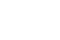It consist of a static tube which measure the static pressure and the pilot tube that measures the total pressure. Total pressure is the sum of static and dynamic pressure.Example 19

A pilot static tube fitted with a pressure gauge is used to measure the speed of the boat at the sea. Given that the speed of the boat does not exceed 10m-1 and the density of seawater is 1050kgm-3. Calculate the maximum pressure of the gauge.

SolutionExample 20

Water flowing in a pipe on aground with velocity 8ms-1 and a gauge pressure of 2 x 105Pa is pumped in a water tank 10m above the ground. Calculate the velocity with which water enters the tank at pressure of 1 x 105PaSolutionExperiment to determine the flow velocity using a pilot-static tube

A liquid of know density, ρ, is allowed to flow in the pilot static tube until the liquid levels are steady in both tubes. The total pressure, Py, is measured from the pilot tube while the static pressure is measured from the static tube.But, Total pressure, Py = static pressure (Px) + dynamic pressureVenturimeterThis consist of the horizontal tube with a constriction at one point. Vertical manometers are inserted into the tube and at the constriction to measure respective pressures. Then## Application of Bernoulli’s principle

1. Origin of the lift force on wings of an aeroplaneThe curved nature of the wings of an aero plane ensures that at the takeoff, the air above the wing has higher velocity than that below. From Bernoulli’s principle, the pressure above the wing is less than that below. This difference in pressure creates a net upward force on the wings.

Example 21

Explain why a spinning ball takes a curved path.

Solution

Air on the upper side is in opposite direction to that of the spin. The resultant velocity of the air is reduced. The air below is in the direction of the spin which increases velocity.

From Bernoulli’s principle, the resultant pressure above the spinning ball is higher than that below it. The difference in pressure creates a net downward force on the ball making it to take on a curve path.

1. Principle of working of a spray1. Pressing the rubber balloon forces the air out of the horizontal tube A at high velocity.
2. From Bernoulli’s principle, this decreases the pressure in the horizontal tube to below atmospheric pressure.
3. The liquid rises up in the vertical tube B from the container.
4. The liquid collides with the fast speeding molecules of air sucked and breaks into fine spray particles.

1. Working of a vacuum cleaner

Example 22

(a) (i) Define coefficient of viscosity and determine its dimensions.

(ii)  the resultant, F, on a steel ball bearing of radius, r, falling with speed, V, a liquid of viscosity, η, is given by F = KηrV, where K is a constant. Show that K is dimensionless.

(b) Write down Bernoulli’s equation for fluid flow, defining all symbols used.

(c) A venturimeter consists of a horizontal tube with a constriction which replaces part of the piping system as shown below.If the cross sectional area of the main pipe is 5.81 x 10-3 m2 and that of the constriction is 2.58 x 10-3 m2. Find the velocity V1 of the liquid in the main pipe

(d) Explain the origin of the lift on an aeroplane at takeoff.

SolutionExample 23

Explain why the acceleration of a ball bearing falling through a liquid decreases continuously until it becomes zero.Solution

For a ball bearing falling through a liquid, it has three forces acting on it namely the up thrust, U, the weight of the bearing, mg, and viscous force, v, acting as shown above.

Viscous force, v, increases with velocity reducing the accelerating force, F = mg – (U+v) which reduces the acceleration to zero when mg = (U +v).

## Compiled by Dr. Bbosa Science

CATEGORIES
•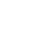•